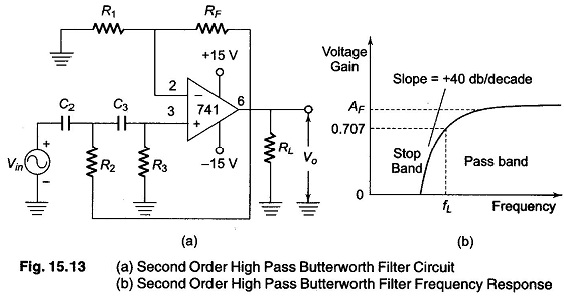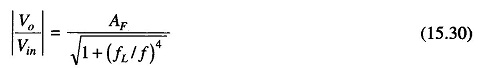## Second Order High Pass Butterworth Filter Derivation:

As in the case of a first order filter, a second order high pass filter can be formed from a second order low pass filter by simply interchanging the fre­quency determining resistance and capacitor. Figure 15.13 (a) shows a Second Order High Pass Butterworth Filter Derivation.The voltage gain magnitude equation of the second order high pass filter is given bywhere

• AF = 1.586 = pass band gain for the second order Butterworth response
• f = frequency of the input signal in Hertz.
• fL = low cutoff frequency

This voltage gain equation can be mathematically determined by using Eq. (15.27), and replacing Z1 and Z2 by capacitors and Z3 and Z4 by resistors.

Scroll to Top